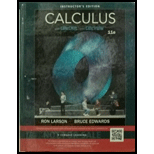Chapter 2.3, Problem 30E### Calculus (MindTap Course List)

11th Edition
Ron Larson + 1 other
ISBN: 9781337275347

#### Solutions

Chapter
Section### Calculus (MindTap Course List)

11th Edition
Ron Larson + 1 other
ISBN: 9781337275347
Textbook Problem

# Finding a Derivative In Exercises 29-40, Find the derivative of the algebraic function. f ( x ) = x 2 + 5 x + 6 x 2 − 4

To determine

To calculate: The derivative of the algebraic function f(x)=x2+5x+6x24.

Explanation

Given:

The function is f(x)=x2+5x+6x24.

Formula used:

The Quotient Rule states that,

(vg)=gvvgg2

The power rule of differentiation states that

ddxxn=nxn1

Calculation:

Consider the function,

f(x)=x2+5x+6x24

Recall the quotient rule,

(vg)=gvvgg2

Let v(x)=x2+5x+6 and g(x)=x24,

Substitute the value of v and g in the quotient rule and differentiate the function with respect to x,

f(x)=(x24)(ddx(x2+5x+6))(ddx(x24))(x2+5x+6)(x24)2=(x24)(2x+5)2x(

### Still sussing out bartleby?

Check out a sample textbook solution.

See a sample solution

#### The Solution to Your Study Problems

Bartleby provides explanations to thousands of textbook problems written by our experts, many with advanced degrees!

Get Started

## Additional Math Solutions

#### Find more solutions based on key concepts